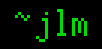## 15-Aug-2010

### P vs. NP

Filed under: humor, math — jlm @ 14:37

So, Vinay Deolalikar has a purported proof that P ≠ NP here. But there must be some error, because I just came up with this simple proof that P = NP !
(If you think “joke proof” sounds like an oxymoron, you can stop reading here.)

Let W(m) ➙ s be a member of NP.
Let X(m; n) ➙ s be the first n symbols of W(m). X is obviously in NP.
So, X has a verifier V(m; n; s) in P.
We can find the first symbol t1 of an output of W(m) by trying V(m; 1; c1), V(m; 1; c2), … for all the symbols ci in the alphabet.
We can find the second symbol t2 by trying V(m; 2; t1c1), V(m; 2; t1c2), etc.
And so on for all the symbols t3, t4, … in the output of W(m).
Finding each symbol takes c calls to V, where c is the alphabet size, a constant. Each call of V takes polynomial time, so we can calculate each individual symbol in polynomial time.
The length of the output of W(m) is necessarily polynomial in ∥m∥, so we have a polynomial number of symbols, each calculable in polynomial time, so we can calculate the entirety of W(m) in polynomial time, so W ∈ P.
P=NP. QED